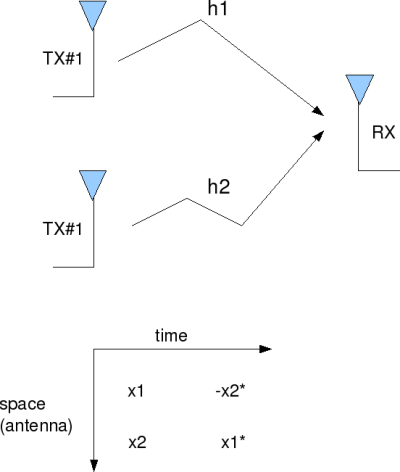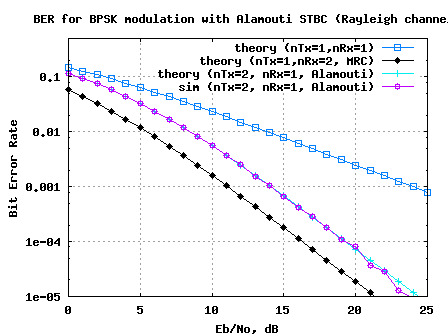- DSP log - http://www.dsplog.com -

Alamouti STBC

Posted By Krishna Sankar On October 16, 2008 @ 6:16 am In MIMO | 273 Comments

In the recent past, we have discussed three receive diversity schemes – Selection combining , Equal Gain Combining  and Maximal Ratio Combining . All the three approaches used the antenna array at the receiver to improve the demodulation performance, albeit with different levels of complexity. Time to move on to a transmit diversity scheme where the information is spread across multiple antennas at the transmitter. In this post, lets discuss a popular transmit diversity scheme called Alamouti Space Time Block Coding (STBC). For the discussion, we will assume that the channel is a flat fading Rayleigh multipath channel  and the modulation is BPSK.

## Alamouti STBC

A simple Space Time Code, suggested by Mr. Siavash M Alamouti in his landmark October 1998 paper – A Simple Transmit Diversity Technique for Wireless Communication , offers a simple method for achieving spatial diversity with two transmit antennas. The scheme is as follows:

1. Consider that we have a transmission sequence, for example

2. In normal transmission, we will be sending in the first time slot, in the second time slot, and so on.

3. However, Alamouti suggested that we group the symbols into groups of two. In the first time slot, send and from the first and second antenna. In second time slot send and from the first and second antenna. In the third time slot send and from the first and second antenna.In fourth time slot, send and from the first and second antenna and so on.

4. Notice that though we are grouping two symbols, we still need two time slots to send two symbols. Hence, there is no change in the data rate.

5. This forms the simple explanation of the transmission scheme with Alamouti Space Time Block coding.Figure: 2-Transmit, 1-Receive Alamouti STBC coding

## Other Assumptions

1. The channel is flat fading – In simple terms, it means that the multipath channel has only one tap. So, the convolution operation reduces to a simple multiplication. For a more rigorous discussion on flat fading and frequency selective fading, may I urge you to review Chapter 15.3 Signal Time-Spreading from [DIGITAL COMMUNICATIONS: SKLAR]2. The channel experience by each transmit antenna is independent from the channel experienced by other transmit antennas.

3. For the transmit antenna, each transmitted symbol gets multiplied by a randomly varying complex number . As the channel under consideration is a Rayleigh channel, the real and imaginary parts of are Gaussian distributed having mean and variance .

4. The channel experienced between each transmit to the receive antenna is randomly varying in time. However, the channel is assumed to remain constant over two time slots.

5. On the receive antenna, the noise has the Gaussian probability density function with

with and .

7. The channel is known at the receiver.

In the first time slot, the received signal is,

.

In the second time slot, the received signal is,

.

where

, is the received symbol on the first and second time slot respectively,
is the channel from transmit antenna to receive antenna,
is the channel from transmit antenna to receive antenna,
, are the transmitted symbols and
is the noise on time slots.

Since the two noise terms are independent and identically distributed,

.

For convenience, the above equation can be represented in matrix notation as follows:

.

Let us define . To solve for , we know that we need to find the inverse of .

We know, for a general m x n matrix, the pseudo inverse  is defined as,

.

The term,

. Since this is a digonal matrix, the inverse is just the inverse of the diagonal elements, i.e

.

The estimate of the transmitted symbol is,

.

If you compare the above equation with the estimated symbol following equalization in Maximal Ratio Combining , you can see that the equations are identical.

## BER with Almouti STBC

Since the estimate of the transmitted symbol with the Alamouti STBC scheme is identical to that obtained from MRC, the BER with above described Alamouti scheme should be same as that for MRC. However, there is a small catch.

With Alamouti STBC, we are transmitting from two antenna’s. Hence the total transmit power in the Alamouti scheme is twice that of that used in MRC. To make the comparison fair, we need to make the total trannsmit power from two antennas in STBC case to be equal to that of power transmitted from a single antenna in the MRC case. With this scaling, we can see that BER performance of 2Tx, 1Rx Alamouti STBC case has a roughly 3dB poorer performance that 1Tx, 2Rx MRC case.

From the post on Maximal Ratio Combining , the bit error rate for BPSK modulation in Rayleigh channel with 1 transmit, 2 receive case is,

, where

.

With Alamouti 2 transmit antenna, 1 receive antenna STBC case,

and Bit Error Rate is

.

## Key points

The fact that is a diagonal matrix ensured the following:
1. There is no cross talk between , after the equalizer.

2. The noise term is still white.

.

## Simulation Model

The Matlab/Octave script performs the following

(a) Generate random binary sequence of +1′s and -1′s.

(b) Group them into pair of two symbols

(c) Code it per the Alamouti Space Time code, multiply the symbols with the channel and then add white Gaussian noise.

(e) Perform hard decision decoding and count the bit errors

(f) Repeat for multiple values of and plot the simulation and theoretical results.Figure: BER plot for BPSK in Rayleigh channel with 2 Transmit and 1 Receive Alamouti STBC

## Observations

Compared to the BER plot for nTx=1, nRx=2 Maximal ratio combining, we can see the Alamouti Space Time Block Coding has around 3dB poorer performance.

## Reference

[DIG-COMM-BARRY-LEE-MESSERSCHMITT] Digital Communication: Third Edition, by John R. Barry, Edward A. Lee, David G. MesserschmittA  Simple Transmit Diversity Technique for Wireless Communication Siavash M Alamouti, IEEE Journal on selected areas in Communication, Vol 16, No, 8, October 1998

URL to article: http://www.dsplog.com/2008/10/16/alamouti-stbc/

URLs in this post:

 Equal Gain Combining: http://www.dsplog.com/2008/09/19/equal-gain-combining/

 Maximal Ratio Combining: http://www.dsplog.com/2008/09/28/maximal-ratio-combining/

 Rayleigh multipath channel: http://www.dsplog.com/2008/07/14/rayleigh-multipath-channel/

 Simple Transmit Diversity Technique for Wireless Communication: http://ieeexplore.ieee.org/iel4/49/15739/00730453.pdf

 pseudo inverse: http://planetmath.org/encyclopedia/Pseudoinverse.html

 Maximal Ratio Combining: http://www.dsplog.com/2008/09/28/maximal-ratio-combining/#MRC

 Maximal Ratio Combining: http://www.dsplog.com/2008/09/28/maximal-ratio-combining

 Matlab/Octave script for simulating BER for 2 transmit, 1 receive Alamouti STBC coding for BPSK modulation in Rayleigh fading channel: http://www.dsplog.com/db-install/wp-content/uploads/2008/10/script_ber_alamouti_stbc_code_bpsk_rayleigh_channel.m